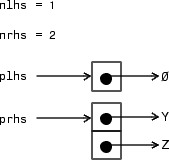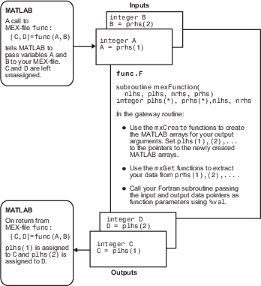Data Flow in Fortran MEX Files

Showing Data Input and Output

Suppose that your MEX file myFunction has two input arguments and one output argument. The MATLAB® syntax is [X] = myFunction(Y, Z). To call myFunction from MATLAB, type:

X = myFunction(Y, Z);

The MATLAB interpreter calls mexFunction, the gateway routine to myFunction, with these arguments:Your input is prhs, a two-element array (nrhs = 2). The first element is a pointer to an mxArray named Y and the second element is a pointer to an mxArray named Z.

Your output is plhs, a one-element array (nlhs = 1) where the single element is a null pointer. The parameter plhs points at nothing because the output X is not created until the subroutine executes.

The gateway routine creates the output array and sets a pointer to it in plhs. If the routine does not assign a value to plhs but you assign an output value to the function when you call it, MATLAB generates an error.

Note

It is possible to return an output value even if nlhs = 0, which corresponds to returning the result in the ans variable.

Gateway Routine Data Flow Diagram

This MEX Cycle diagram shows how inputs enter a MEX file, what functions the gateway routine performs, and how outputs return to MATLAB.

In this example, the syntax of the MEX file func is [C, D] = func(A,B). In the figure, a call to func tells MATLAB to pass variables A and B to your MEX file. C and D are left unassigned.

The gateway routine uses the mxCreate* functions to create the MATLAB arrays for your output arguments. It sets plhs and plhs to the pointers to the newly created MATLAB arrays. It uses the mxGet* functions to extract your data from your input arguments prhs and prhs. Finally, it calls your computational routine, passing the input and output data pointers as function parameters.

MATLAB assigns plhs to C and plhs to D.

Fortran MEX Cycle